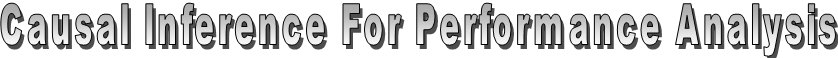This research investigates how causal structure learning algorithms can aid the performance analysis of (parallel) programs.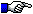Our causal learning moduleExperimental SetupResearch results, explained with examplesInformation analysis moduleKernel density estimation (KDE) moduleInteractive tutorial on causality, information and KDE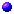Introduction

Although causality is still a highly debated issue among statisticians, we believe it provides a natural and intuitive representation about the relations among variables. We propose the use of causal models for modeling how variables influence the overall performance of (parallel) applications.
The following figure represents a simplified performance model of a sort algorithm: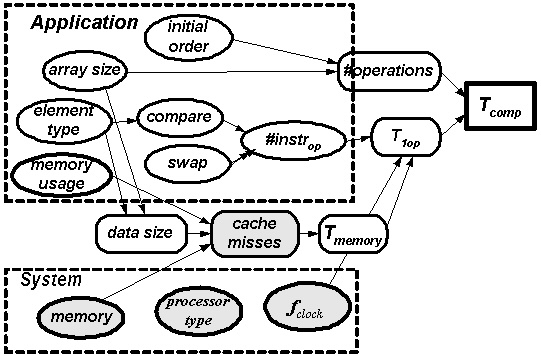It contains application parameters (at the left), system parameters (at the bottom), the overall performance (at the right) and intermediate variables that can be measured inside the application of the system.

The following figure shows the basic causal model of the performance of a parallel application: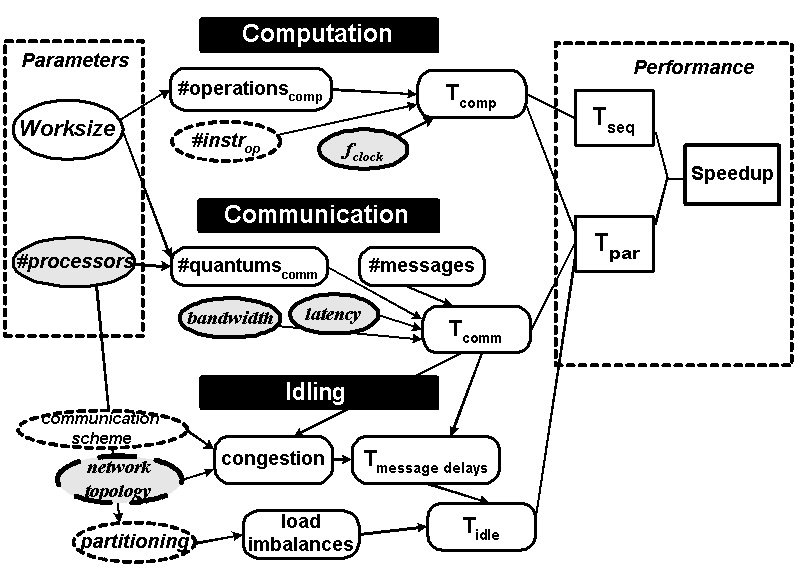This model is reflected in the analysis of EPPA.Causal Structure Learning

Besides the formalization of causality by Judea Pearl, another important scientific advance was the invention of algorithms that can learn causal models from observational data. The research of Spirtes, Glymour and Scheines (SGS) has resulted in the TETRAD tool. Our work is based on TETRAD's algorithms, which we extended to capture the full complexity of performance models:
1. A mixture of continuous and discrete data.
2. A form-free measure of dependency.
3. The handling of deterministic relations.
4. Context-specific variables and independencies.
The first two problems are solved by using the mutual information as dependency measure, which is based on Shannon's entropy of distributions. Kernel Density Estimation is a technique for deriving a distribution from experimental data. The development was conducted by Frederik Verbist.
The handling of deterministic relations is attained by considering the case that two variables have equivalent information about a third variable. The only objective criterion for deciding upon which variables are directly related is then the complexity of the relations. This work is done by Jan Lemeire. The last extension is currently being studied by Joris Borms, he will use context-specific graphs.Our causal learning moduleResearch results, explained with examplesPhilosophy of Learning, Minimum Description Length & Assumptions

One of the hardest criticisms of causality and the learning algorithms are the assumptions that are made.
We want to answer by considering learning in general, in a philosophical way: what can we learn from observations? It is clear that no method can guarantee that the 'real' model will be learnt.
An interesting side question is if the real, physical world can be known. After all, everything we know about the world comes from observation (and partly from a book). See the opinions from Jorma Rissanen and Peter Grünwald about this.
By linking the work of causal structure learning with the ideas developed by the field of Algorithmic Information Theory (see Paul Vitanyi) around regularities (= compression!) and typicalness (randomness = incompressibility), I believe that we an conclude that we have to search for regularities in data and construct models that capture these regularities. Moreover, causal models suggest that we have to look for models that capture all regularities!Draft paper Causal Models as Minimal Descriptions of Multivariate Systems.

last updated: March, 10th 2006 by Jan Lemeire
Parallel Computing Lab, VUB